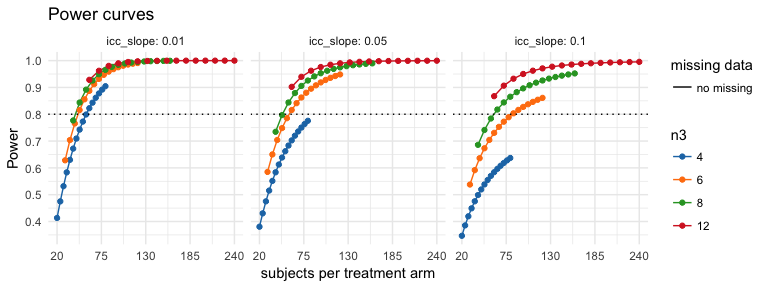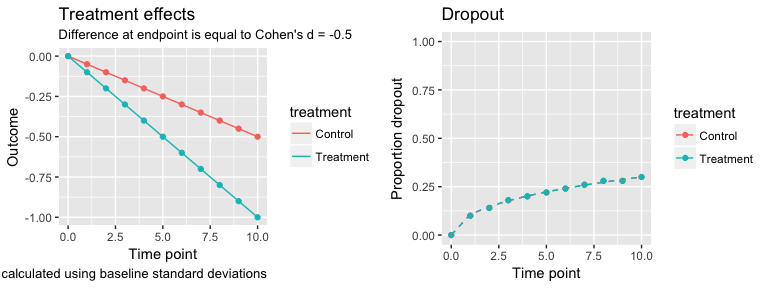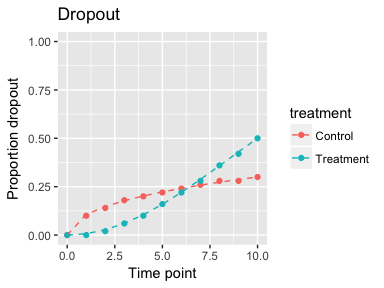# Power Analysis for Three-level Longitudinal Models with Missing Data

#### 2017-09-11

This vignette shows how to setup and calculate power for three-level models with nesting in one or both of the treatment arms.

# Three-level models

Currently, powerlmm support three-level models that are fully nested or partially nested; crossed designs are not yet supported.

## Model formulation

In standard multilevel notation the fully nested three-level model is \begin{align} \text{Level 1}& \notag\\\ Y_{ijk} &= \beta_{0jk} + \beta_{1jk}t_{ijk} + R_{ijk}\\\ \text{Level 2}& \notag\\\ \beta_{0jk} &= \gamma_{00k} + U_{0jk} \\\ \beta_{1jk} &= \gamma_{10k} + U_{1jk} \\\ \text{Level 3}& \notag\\\ \gamma_{00k} &= \delta_{000} + \delta_{001} TX_k + V_{0k} \\\ \gamma_{10k} &= \delta_{100} + \delta_{101} TX_k + V_{1k} \\\ \end{align} with, $\begin{equation} \begin{pmatrix} U_{0j} \\\ U_{1j} \end{pmatrix} \sim\mathcal{N} \left( \begin{matrix} 0 &\\\ 0 \end{matrix} , \begin{matrix} \sigma_{u_0}^2 & \sigma_{u_{01}} \\\ \sigma_{u_{01}} & \sigma_{u_{1}}^2 \end{matrix} \right) , \end{equation}$ and, $\begin{equation} \begin{pmatrix} V_{0k} \\\ V_{1k} \end{pmatrix} \sim\mathcal{N} \left( \begin{matrix} 0 &\\\ 0 \end{matrix} , \begin{matrix} \sigma_{v_0}^2 & \sigma_{v_{01}} \\\ \sigma_{v_{01}} & \sigma_{v_{1}}^2 \end{matrix} \right) , \end{equation}$ and $\begin{equation} R_{ijk} \sim\mathcal{N}(0, ~\sigma^2) \end{equation}$

The corresponding arguments in study_parameters are

parameter study_parameters()-argument
$$\delta_{000}$$ fixed_intercept
$$\delta_{001}$$ NA; assumed to be 0
$$\delta_{100}$$ fixed_slope
$$\delta_{101}$$ calculated from cohend; standardized using baseline standard deviation
$$\sigma_{u_0}$$ sigma_subject_intercept
$$\sigma_{u_1}$$ sigma_subject_slope
$$\sigma_{u_{01}}$$ calculated from cor_subject
$$\sigma_{v_0}$$ sigma_cluster_intercept
$$\sigma_{v_1}$$ sigma_cluster_slope
$$\sigma_{v_{01}}$$ calculated from cor_cluster
$$\sigma$$ sigma_error

## Standardized and unstandardized formulation

If you are only interested in power of a model, then it is not necessary to define all parameters in the model. For a three-level model power depends on n1, n2, n3, the amount of baseline variance at the subject level (icc_pre_subjects) and the ratio of subject-level random slope variance to the within-subject error variance (var_ratio), and the amount of random slope variance at the third-level (icc_slope). Power is also influenced by the ratio of the baseline variances (icc_pre_subjects, icc_pre_clusters), since the standardization of Cohen’s d depends on those parameters.

The relative standardized inputs are calculated in the following way.

Standardized Calculation
icc_pre_subjects $$\sigma_{u_0}^2/(\sigma_{u_0}^2 + \sigma_{v_0}^2 + \sigma^2)$$
icc_pre_clusters $$\sigma_{v_0}^2/(\sigma_{u_0}^2 + \sigma_{v_0}^2 + \sigma^2)$$
icc_slope $$\sigma_{v_1}^2/(\sigma_{u_1}^2 + \sigma_{v_1}^2)$$
var_ratio $$(\sigma_{u_1}^2 + \sigma_{v_1}^2)/\sigma^2$$
cohend $$\gamma_{11}/(\sigma_{u_0}^2 + \sigma_{v_0}^2 + \sigma^2)$$

Using the argument names in study_parameters, the standardized inputs are calculated in the following way.

Standardized Calculation
icc_pre_subjects sigma_subject_intercept^2/(sigma_subject_intercept^2 + sigma_cluster_intercept^2 + sigma_error^2)
icc_pre_clusters sigma_cluster_intercept^2/(sigma_subject_intercept^2 + sigma_cluster_intercept^2 + sigma_error^2)
icc_slope sigma_custer_slope^2/(sigma_cluster_slope^2 + sigma_subject_slope^2)
var_ratio (sigma_subject_slope^2 + sigma_custer_slope^2)/sigma_error^2
cohend slope_diff/(sigma_subject_intercept^2 + sigma_cluster_intercept^2 + sigma_error^2)

# Study setup

This example will show how to calculate power for the time:treatment-interaction. We assume that the two treatment arms will differ by a Cohen’s d of 0.8 at post-test. The treatment period is 11 weeks with weekly measures, and each treatment group has 4 therapists that each treat 10 participants. We are expecting that 5 % of the total slope variance will be at the therapist level, and there will be a moderate amount of heterogeneity in change over time (var_ratio = 0.02), Lastly, 50 % of the baseline variance is at the subject level, and icc_pre_cluster is set to 0 since we are randomizing subjects to clusters, and hence there should be no therapist effect at baseline.

p <- study_parameters(n1 = 11,
n2 = 10,
n3 = 4,
icc_pre_subject = 0.5,
icc_pre_cluster = 0,
icc_slope = 0.05,
var_ratio = 0.02,
cohend = -0.8)
p
##
##      Study setup (three-level)
##
##               n1 = 11
##               n2 = 10 (treatment)
##                    10 (control)
##               n3 = 4  (treatment)
##                    4  (control)
##                    8  (total)
##          total_n = 40 (treatment)
##                    40 (control)
##                    80 (total)
##          dropout = No missing data
## icc_pre_subjects = 0.5
## icc_pre_clusters = 0
##        icc_slope = 0.05
##        var_ratio = 0.02
##           cohend = -0.8

## Power

To calcalute power for the study, we use the get_power-method using the object created with study_parameters.

get_power(p)
##
##      Power calculation for longitudinal linear mixed model (three-level)
##                            with missing data and unbalanced designs
##
##               n1 = 11
##               n2 = 10 (treatment)
##                    10 (control)
##               n3 = 4  (treatment)
##                    4  (control)
##                    8  (total)
##          total_n = 40 (treatment)
##                    40 (control)
##                    80 (total)
##          dropout = No missing data
## icc_pre_subjects = 0.5
## icc_pre_clusters = 0
##        icc_slope = 0.05
##        var_ratio = 0.02
##           cohend = -0.8
##            power = 0.58

## Understanding the model

Several helper functions are available to help you explore the implications of the model’s parameters. For instance, we can calculate the variance partitioning coefficients (VPC), to see how the proportion of variance at each level change over time.

get_VPC(p)
## # Percentage (%) of total variance at each level and time point
##    time between_clusters between_subjects within_subjects tot_var
## 1     0             0.00               50              50       0
## 2     1             0.05               50              50       1
## 3     2             0.19               52              48       4
## 4     3             0.41               54              46       9
## 5     4             0.69               56              43      16
## 6     5             1.00               59              40      25
## 7     6             1.32               62              37      36
## 8     7             1.64               65              34      49
## 9     8             1.95               68              30      64
## 10    9             2.24               70              28      81
## 11   10             2.50               72              25     100

between_clusters/100 corresponds to the ICC at level 3 per time point, i.e. the correlation between two subjects belonging to the same cluster. tot_var gives the percentage change in variance per time point, compared to the baseline total variance, i.e. at the last time point the total variance is 100 % larger than at baseline. If you want to see the correlation between time points, you can use get_correlation_matrix(p)

get_correlation_matrix(p)
##       0    1    2    3    4    5    6    7    8    9   10
## 0  1.00 0.50 0.49 0.48 0.46 0.45 0.43 0.41 0.39 0.37 0.35
## 1  0.50 1.00 0.51 0.51 0.50 0.49 0.48 0.46 0.45 0.44 0.42
## 2  0.49 0.51 1.00 0.53 0.53 0.53 0.52 0.51 0.51 0.50 0.49
## 3  0.48 0.51 0.53 1.00 0.55 0.56 0.56 0.56 0.55 0.55 0.54
## 4  0.46 0.50 0.53 0.55 1.00 0.58 0.59 0.59 0.59 0.59 0.59
## 5  0.45 0.49 0.53 0.56 0.58 1.00 0.61 0.62 0.63 0.63 0.63
## 6  0.43 0.48 0.52 0.56 0.59 0.61 1.00 0.65 0.66 0.66 0.67
## 7  0.41 0.46 0.51 0.56 0.59 0.62 0.65 1.00 0.68 0.69 0.70
## 8  0.39 0.45 0.51 0.55 0.59 0.63 0.66 0.68 1.00 0.71 0.72
## 9  0.37 0.44 0.50 0.55 0.59 0.63 0.66 0.69 0.71 1.00 0.74
## 10 0.35 0.42 0.49 0.54 0.59 0.63 0.67 0.70 0.72 0.74 1.00

Lastly, a longitudinal study with random slopes implies that the standard deviation per time point follows a quadratic function over time. You can get the model implied standard deviations per time point using get_sds.

get_sds(p)
##    time SD_with_random_slopes SD_no_cluster_random_slope
## 1     0                   1.0                        1.0
## 2     1                   1.0                        1.0
## 3     2                   1.0                        1.0
## 4     3                   1.0                        1.0
## 5     4                   1.1                        1.1
## 6     5                   1.1                        1.1
## 7     6                   1.2                        1.2
## 8     7                   1.2                        1.2
## 9     8                   1.3                        1.3
## 10    9                   1.3                        1.3
## 11   10                   1.4                        1.4
##    SD_no_random_slopes
## 1                    1
## 2                    1
## 3                    1
## 4                    1
## 5                    1
## 6                    1
## 7                    1
## 8                    1
## 9                    1
## 10                   1
## 11                   1

Since, we defined our study using only standardized inputs, get_sds tells us by what magnitude the SDs change over time. At week the SDs will be 1.4 times larger than at baseline. If we used raw values for all parameters defined with study_parameters, then these SDs would be the actual model implied standard deviations per time points. Its useful to check that these values make sense.

# Compare different values

In a three-level design with random slopes, power depends heavily on the amount of clusters, and icc_slope and var_ratio. The function get_power_table is helpful when you want to compare the effect of up to 3 different parameters.

library(ggplot2)
x <- get_power_table(p, n2 = 5:20, n3 = c(4, 6, 8, 12), icc_slope = c(0.01, 0.05, 0.1))
plot(x) + scale_x_continuous(breaks = seq(20, 240, length.out = 5))The plot shows clearly that including more clusters yields higher power for the same amount of total participants.

# Unbalanced designs

It is possible to define different cluster size both within a treatment and between treatment groups using the per_treatment function. Here’s an example of how to have more clusters in the treatment group.

p <- study_parameters(n1 = 11,
n2 = 10,
n3 = per_treatment(control = 2,
treatment = 10),
icc_pre_subject = 0.5,
icc_pre_cluster = 0,
icc_slope = 0.05,
var_ratio = 0.02,
cohend = -0.8)
p
##
##      Study setup (three-level)
##
##               n1 = 11
##               n2 = 10  (treatment)
##                    10  (control)
##               n3 = 10  (treatment)
##                    2   (control)
##                    12  (total)
##          total_n = 100 (treatment)
##                    20  (control)
##                    120 (total)
##          dropout = No missing data
## icc_pre_subjects = 0.5
## icc_pre_clusters = 0
##        icc_slope = 0.05
##        var_ratio = 0.02
##           cohend = -0.8

Since both treatment arms have the same amount of participants per cluster (n2), the treatment arm will have much more participants. However, we can also use per_treatment to set the number of participants per cluster to be different in the two treatment arms.

p <- study_parameters(n1 = 11,
n2 = per_treatment(control = 10,
treatment = 2),
n3 = per_treatment(control = 2,
treatment = 10),
icc_pre_subject = 0.5,
icc_pre_cluster = 0,
icc_slope = 0.05,
var_ratio = 0.02,
cohend = -0.8)
p
##
##      Study setup (three-level)
##
##               n1 = 11
##               n2 = 2  (treatment)
##                    10 (control)
##               n3 = 10 (treatment)
##                    2  (control)
##                    12 (total)
##          total_n = 20 (treatment)
##                    20 (control)
##                    40 (total)
##          dropout = No missing data
## icc_pre_subjects = 0.5
## icc_pre_clusters = 0
##        icc_slope = 0.05
##        var_ratio = 0.02
##           cohend = -0.8

In this example, both treatment arms have 20 participants in total, but different amount of clusters, e.g. therapists.

## Different cluster sizes within a treatment arm

Lastly, we can use the unequal_clusters-function to define clusters of varying sizes within a treatment arm.

p <- study_parameters(n1 = 11,
n2 = unequal_clusters(2, 5, 10, 30),
icc_pre_subject = 0.5,
icc_pre_cluster = 0,
icc_slope = 0.05,
var_ratio = 0.02,
cohend = -0.8)
p
##
##      Study setup (three-level)
##
##               n1 = 11
##               n2 = 2, 5, 10, 30 (treatment)
##                    2, 5, 10, 30 (control)
##               n3 = 4            (treatment)
##                    4            (control)
##                    8            (total)
##          total_n = 47           (treatment)
##                    47           (control)
##                    94           (total)
##          dropout = No missing data
## icc_pre_subjects = 0.5
## icc_pre_clusters = 0
##        icc_slope = 0.05
##        var_ratio = 0.02
##           cohend = -0.8

Here we have setup a study that have 4 therapists (clusters) per treatment arm, but each therapist are assigned a different amount of subjects (2, 5, 10 and 30 clients, respectively). When we use unequal_clusters we don’t need to specify n3. Moreover, unequal_clusters can be combined with per_treatment, like so:

n2 <- per_treatment(control = unequal_clusters(5, 10, 15),
treatment = unequal_clusters(2, 3, 5, 5, 10, 15, 25))

p <- study_parameters(n1 = 11,
n2 = n2,
icc_pre_subject = 0.5,
icc_pre_cluster = 0,
icc_slope = 0.05,
var_ratio = 0.02,
cohend = -0.8)
p
##
##      Study setup (three-level)
##
##               n1 = 11
##               n2 = 2, 3, 5, 5, 10, 15, 25 (treatment)
##                    5, 10, 15              (control)
##               n3 = 7                      (treatment)
##                    3                      (control)
##                    10                     (total)
##          total_n = 65                     (treatment)
##                    30                     (control)
##                    95                     (total)
##          dropout = No missing data
## icc_pre_subjects = 0.5
## icc_pre_clusters = 0
##        icc_slope = 0.05
##        var_ratio = 0.02
##           cohend = -0.8

# Partially nesting

Partially nesting is common in e.g. clinical psychology studies, when a treatment is compared to a wait-list control. The wait-list arm will only have two levels, but the treatment arm will have three-levels, just as in the previous examples. To define a partially nested study you just need to add partially_nested = TRUE.

p <- study_parameters(n1 = 11,
n2 = unequal_clusters(2, 5, 10, 30),
icc_pre_subject = 0.5,
icc_pre_cluster = 0,
icc_slope = 0.05,
var_ratio = 0.02,
partially_nested = TRUE,
cohend = -0.8)

p
##
##      Study setup (three-level, partially nested)
##
##               n1 = 11
##               n2 = 2, 5, 10, 30 (treatment)
##                    47           (control)
##               n3 = 4            (treatment)
##                    0            (control)
##                    4            (total)
##          total_n = 47           (treatment)
##                    47           (control)
##                    94           (total)
##          dropout = No missing data
## icc_pre_subjects = 0.5
## icc_pre_clusters = 0
##        icc_slope = 0.05
##        var_ratio = 0.02
##           cohend = -0.8

No matter how you define n2 and n3, for a partially nested design n2 will automatically be set to the total amount of subjects. Here’s two examples

p1 <- study_parameters(n1 = 11,
n2 = 5,
n3 = 5,
icc_pre_subject = 0.5,
icc_pre_cluster = 0,
icc_slope = 0.05,
var_ratio = 0.02,
partially_nested = TRUE,
cohend = -0.8)

p2 <- study_parameters(n1 = 11,
n2 = per_treatment(control = 50,
treatment = 5),
n3 = per_treatment(control = 1,
treatment = 5),
icc_pre_subject = 0.5,
icc_pre_cluster = 0,
icc_slope = 0.05,
var_ratio = 0.02,
partially_nested = TRUE,
cohend = -0.8)

p1
##
##      Study setup (three-level, partially nested)
##
##               n1 = 11
##               n2 = 5  (treatment)
##                    25 (control)
##               n3 = 5  (treatment)
##                    0  (control)
##                    5  (total)
##          total_n = 25 (treatment)
##                    25 (control)
##                    50 (total)
##          dropout = No missing data
## icc_pre_subjects = 0.5
## icc_pre_clusters = 0
##        icc_slope = 0.05
##        var_ratio = 0.02
##           cohend = -0.8
p2
##
##      Study setup (three-level, partially nested)
##
##               n1 = 11
##               n2 = 5  (treatment)
##                    50 (control)
##               n3 = 5  (treatment)
##                    0  (control)
##                    5  (total)
##          total_n = 25 (treatment)
##                    50 (control)
##                    75 (total)
##          dropout = No missing data
## icc_pre_subjects = 0.5
## icc_pre_clusters = 0
##        icc_slope = 0.05
##        var_ratio = 0.02
##           cohend = -0.8

# Missing data

Missing data can be accounted for in the power calculations by the argument dropout, which can be added to all the available designs. The missing data is assumed to be monotonically increasing, hence intermittent missing data is not currently supported. Two helper functions are used to define the dropout pattern; either dropout_manual or dropout_weibull. Here I will use dropout_weibull.

p <- study_parameters(n1 = 11,
n2 = 10,
n3 = 5,
icc_pre_subject = 0.5,
icc_pre_cluster = 0,
var_ratio = 0.02,
icc_slope = 0.05,
dropout = dropout_weibull(proportion = 0.3,
rate = 1/2),
fixed_slope = -0.5/10,
cohend = -0.5)

p
##
##      Study setup (three-level)
##
##               n1 = 11
##               n2 = 10  (treatment)
##                    10  (control)
##               n3 = 5   (treatment)
##                    5   (control)
##                    10  (total)
##          total_n = 50  (treatment)
##                    50  (control)
##                    100 (total)
##          dropout =  0,  1,  2,  3,  4,  5,  6,  7,  8,  9, 10 (time)
##                     0, 11, 15, 18, 20, 22, 24, 26, 27, 29, 30 (%, control)
##                     0, 11, 15, 18, 20, 22, 24, 26, 27, 29, 30 (%, treatment)
## icc_pre_subjects = 0.5
## icc_pre_clusters = 0
##        icc_slope = 0.05
##        var_ratio = 0.02
##           cohend = -0.5

Here I’ve chosen to have a total of 30 % of the participant dropout during the study, with more dropout occurring earlier in the study period. We can plot the model and the missing data pattern using the tplot-method.

plot(p)And the power can be calculated using get_power.

get_power(p)
##
##      Power calculation for longitudinal linear mixed model (three-level)
##                            with missing data and unbalanced designs
##
##               n1 = 11
##               n2 = 10  (treatment)
##                    10  (control)
##               n3 = 5   (treatment)
##                    5   (control)
##                    10  (total)
##          total_n = 50  (treatment)
##                    50  (control)
##                    100 (total)
##          dropout =  0,  1,  2,  3,  4,  5,  6,  7,  8,  9, 10 (time)
##                     0, 11, 15, 18, 20, 22, 24, 26, 27, 29, 30 (%, control)
##                     0, 11, 15, 18, 20, 22, 24, 26, 27, 29, 30 (%, treatment)
## icc_pre_subjects = 0.5
## icc_pre_clusters = 0
##        icc_slope = 0.05
##        var_ratio = 0.02
##           cohend = -0.5
##            power = 0.3

## Different dropout patterns per treatment group

The helper function per_treatment can also be used to define different dropout patterns per treatment arm.

d <- per_treatment(control = dropout_weibull(proportion = 0.3,
rate = 1/2),
treatment = dropout_weibull(proportion = 0.5,
rate = 2))

p2 <- study_parameters(n1 = 11,
n2 = 10,
n3 = 5,
icc_pre_subject = 0.5,
icc_pre_cluster = 0,
var_ratio = 0.02,
icc_slope = 0.05,
dropout = d,
fixed_slope = -0.5/10,
cohend = -0.5)

plot(p2, plot = 2)# The design effect and type I error rates if clustering is ignored

You might be interested in calculation the design effect. The get_DEFT-function will calculate the design effect for the standard error of the time:treatment-interaction. DEFT tells you how many times larger the standard errors from the misspecified model should be. It will also report the approximate type I error if the random slope at the third level is omitted. Here’s an example that investigates the impact of increasing cluster sizes on the DEFT.

p2 <- study_parameters(n1 = 11,
n2 = c(5, 10, 15, 20, 30),
n3 = 5,
icc_pre_subject = 0.5,
icc_pre_cluster = 0,
var_ratio = 0.02,
icc_slope = 0.05,
dropout = dropout_weibull(proportion = 0.3,
rate = 1/2),
fixed_slope = -0.5/10,
cohend = -0.5)
get_DEFT(p2)
##   icc_slope var_ratio     DEFT approx_type1
## 1      0.05      0.02 1.049739   0.06188818
## 2      0.05      0.02 1.110002   0.07744110
## 3      0.05      0.02 1.168033   0.09334643
## 4      0.05      0.02 1.222165   0.10878427
## 5      0.05      0.02 1.325483   0.13922620

We can see that the design effect increases with more subjects per cluster. Even when only 5 % of the slope variance is at the cluster level, type I errors can be considerably inflated if the random slope at the third-level is ignored.